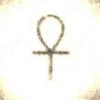Iterated nandXorterFellowPosts: 93 Threads: 30 Joined: Aug 2016 03/12/2017, 03:08 PM I am interested in iterates (and uniterates) of logical operators, like nand, xor ... etc. . Let's investigate the nand op: x↑y = ¬(x&y) Iterating x O y means this: x O x O ... O x (y-times) Thus x ↑ x ↑ ... ↑ x := x ↑↑ y x ↑↑ 1 = x x ↑↑ 2 = x ↑ x = ¬x x ↑↑ 3 = x ↑ ¬x = ¬(x & ¬x) = x v ¬x x ↑↑ 4 = x ↑ (x v ¬x) = ¬(x & (x v ¬x)) = x & ¬x x ↑↑ 5 = x ↑ (x & ¬x) = ¬(x & (x & ¬x)) = x v ¬x x ↑↑ 6 = x & ¬x x ↑↑ 7 = x v ¬x ... etc. So x ↑↑ 2k = x & ¬x x ↑↑ 2k-1 = x v ¬x where k is bigger integer than 2 My question: Can k be any real or complex number? (In my view to do this, we should know what "between" & and v is.) Xorter Unizotommy1729Ultimate FellowPosts: 1,918 Threads: 414 Joined: Feb 2009 03/23/2017, 01:27 PM (03/12/2017, 03:08 PM)Xorter Wrote: I am interested in iterates (and uniterates) of logical operators, like nand, xor ... etc. . Let's investigate the nand op: x↑y = ¬(x&y) Iterating x O y means this: x O x O ... O x (y-times) Thus x ↑ x ↑ ... ↑ x := x ↑↑ y x ↑↑ 1 = x x ↑↑ 2 = x ↑ x = ¬x x ↑↑ 3 = x ↑ ¬x = ¬(x & ¬x) = x v ¬x x ↑↑ 4 = x ↑ (x v ¬x) = ¬(x & (x v ¬x)) = x & ¬x x ↑↑ 5 = x ↑ (x & ¬x) = ¬(x & (x & ¬x)) = x v ¬x x ↑↑ 6 = x & ¬x x ↑↑ 7 = x v ¬x ... etc. So x ↑↑ 2k = x & ¬x x ↑↑ 2k-1 = x v ¬x where k is bigger integer than 2 My question: Can k be any real or complex number? (In my view to do this, we should know what "between" & and v is.) --- I like the basic idea to do dynamics in set theory , Logic and the alike. However i see many issues. First you write alot " x and not x " and "x or not x" and they are ( in boolean ) either trivial or paradoxical ! Like " this sentense is false ". or " this is true and false " , " this is true or false. Secondly suppose we let times -1 mean not. Than iterations of not give the unit circle in the complex plane. but what does it mean " i " ? What does it mean to half-iterate NOT ? 0r the pi th iteration of x OR y ??? you only have a few things like and or not true etc. but you want continue iterations ?? or if you introduce new things like " i " above you need to Well define it !! so as of now im very skeptical for continue iterations. as for integer iterates that might work. i think one then needs to associate it with groups or rings. maybe modular arithmetic too. Regards tommy1729 the masterXorterFellowPosts: 93 Threads: 30 Joined: Aug 2016 03/27/2017, 06:51 PM (03/23/2017, 01:27 PM)tommy1729 Wrote: --- I like the basic idea to do dynamics in set theory , Logic and the alike. However i see many issues. First you write alot " x and not x " and "x or not x" and they are ( in boolean ) either trivial or paradoxical ! Like " this sentense is false ". or " this is true and false " , " this is true or false. Secondly suppose we let times -1 mean not. Than iterations of not give the unit circle in the complex plane. but what does it mean " i " ? What does it mean to half-iterate NOT ? 0r the pi th iteration of x OR y ??? you only have a few things like and or not true etc. but you want continue iterations ?? or if you introduce new things like " i " above you need to Well define it !! so as of now im very skeptical for continue iterations. as for integer iterates that might work. i think one then needs to associate it with groups or rings. maybe modular arithmetic too. Regards tommy1729 the master Very good questions. Well, to be honest, (x or not x), (x and not x) are neither trivial nor paradoxical, just think of fuzzy logic with x=0.5 (half truth or sg like this), then each expression results 0.5, right? What is the half iterative of negation? The imaginary negation, about what we know that: ¬^.5 o ¬^.5 = ¬, where o is composition. It is as mysterious as the imaginary unit. I think. But it solves your so-called paradox, that: "This statement is false.". Its value neither true nor false, it is (¬^.5)(true), so so-called this is the imaginary truth ... solving the all the paradoxes.What is the pi-th and the i-th iterate of the logical ops? This is my question. Xorter Unizo « Next Oldest | Next Newest »

 Possibly Related Threads… Thread Author Replies Views Last Post 4 hypothesis about iterated functions Shanghai46 11 1,417 04/22/2023, 08:22 PM Last Post: Shanghai46 Question about the properties of iterated functions Shanghai46 9 1,115 04/21/2023, 09:07 PM Last Post: Shanghai46 [MSE] iterated sin using Besselfunction 1st kind Gottfried 7 1,358 12/18/2022, 02:06 PM Last Post: Gottfried Iterated function convergence Daniel 1 547 12/18/2022, 01:40 AM Last Post: JmsNxn Uniqueness of fractionally iterated functions Daniel 7 2,117 07/05/2022, 01:21 AM Last Post: JmsNxnIterated Hyperbolic Sine and Iterated Natural Logarithm Catullus 2 1,217 06/11/2022, 11:58 AM Last Post: tommy1729 Generalized Kneser superfunction trick (the iterated limit definition) MphLee 25 16,803 05/26/2021, 11:55 PM Last Post: MphLee iterated derivation Xorter 0 2,851 06/09/2019, 09:43 PM Last Post: Xorter 1st iterated derivatives and the tetration of 0 Xorter 0 3,723 05/12/2018, 12:34 PM Last Post: Xorter Iterated polynomials JmsNxn 4 13,280 12/16/2010, 09:00 PM Last Post: JmsNxn

Users browsing this thread: 1 Guest(s)